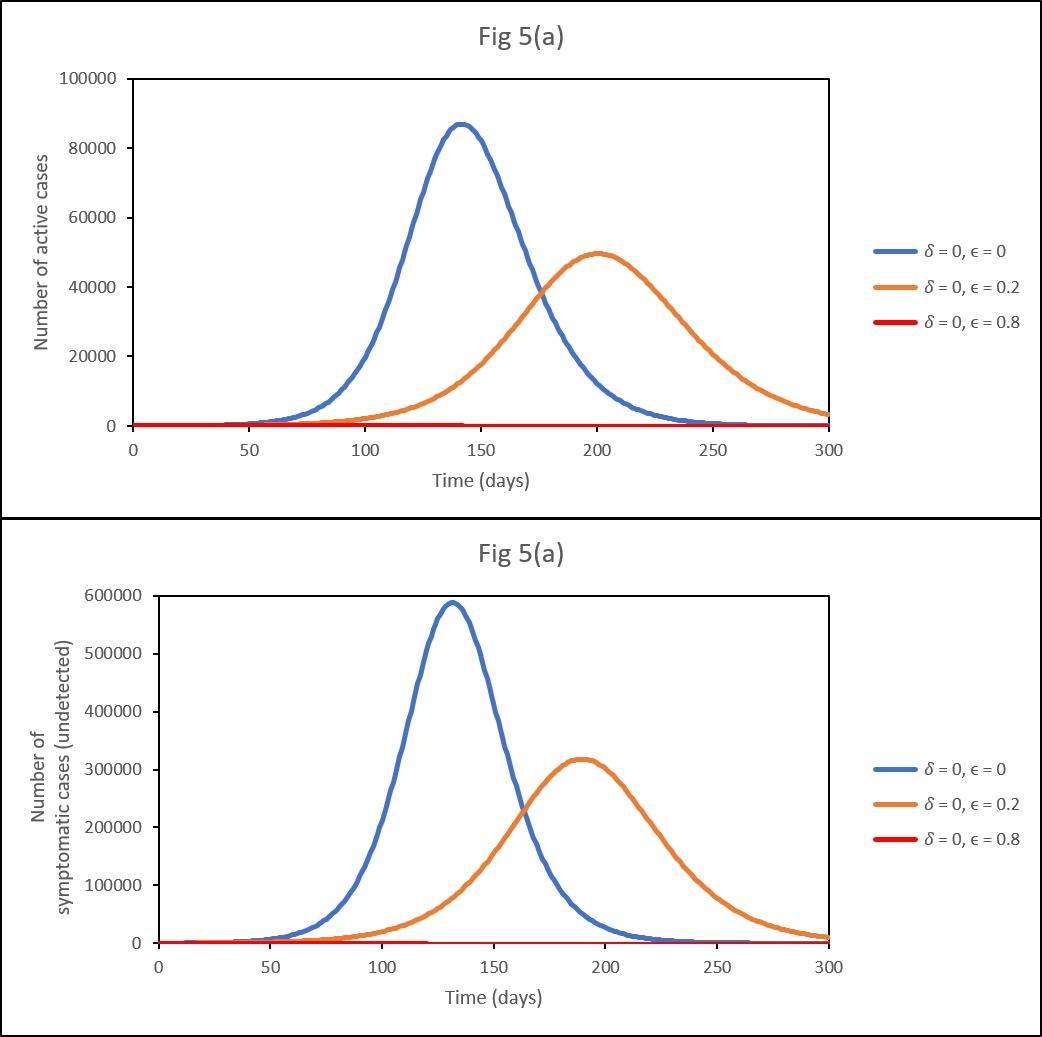## Okuonghae2020 - SEAIR model of COVID-19 transmission in Lagos, NigeriaModel Identifier
BIOMD0000000991
Short description
This work examines the impact of various non-pharmaceutical control measures (government and personal) on the population dynamics of the novel coronavirus disease 2019 (COVID-19) in Lagos, Nigeria, using an appropriately formulated mathematical model. Using the available data, since its first reported case on 16 March 2020, we seek to develop a predicative tool for the cumulative number of reported cases and the number of active cases in Lagos; we also estimate the basic reproduction number of the disease outbreak in the aforementioned State in Nigeria. Using numerical simulations, we show the effect of control measures, specifically the common social distancing, use of face mask and case detection (via contact tracing and subsequent testings) on the dynamics of COVID-19. We also provide forecasts for the cumulative number of reported cases and active cases for different levels of the control measures being implemented. Numerical simulations of the model show that if at least 55% of the population comply with the social distancing regulation with about 55% of the population effectively making use of face masks while in public, the disease will eventually die out in the population and that, if we can step up the case detection rate for symptomatic individuals to about 0.8 per day, with about 55% of the population complying with the social distancing regulations, it will lead to a great decrease in the incidence (and prevalence) of COVID-19.
Format
SBML (L2V4)
Related Publication
• Analysis of a mathematical model for COVID-19 population dynamics in Lagos, Nigeria.• Okuonghae D, Omame A
• Chaos, solitons, and fractals , 10/ 2020 , Volume 139 , pages: 110032 , PubMed ID: 32834593
• Department of Mathematics, University of Benin, Benin City, Nigeria.
• This work examines the impact of various non-pharmaceutical control measures (government and personal) on the population dynamics of the novel coronavirus disease 2019 (COVID-19) in Lagos, Nigeria, using an appropriately formulated mathematical model. Using the available data, since its first reported case on 16 March 2020, we seek to develop a predicative tool for the cumulative number of reported cases and the number of active cases in Lagos; we also estimate the basic reproduction number of the disease outbreak in the aforementioned State in Nigeria. Using numerical simulations, we show the effect of control measures, specifically the common social distancing, use of face mask and case detection (via contact tracing and subsequent testings) on the dynamics of COVID-19. We also provide forecasts for the cumulative number of reported cases and active cases for different levels of the control measures being implemented. Numerical simulations of the model show that if at least 55% of the population comply with the social distancing regulation with about 55% of the population effectively making use of face masks while in public, the disease will eventually die out in the population and that, if we can step up the case detection rate for symptomatic individuals to about 0.8 per day, with about 55% of the population complying with the social distancing regulations, it will lead to a great decrease in the incidence (and prevalence) of COVID-19.
Contributors
Paul Jonas Jost, Tung Nguyen, Kausthubh Ramachandran, Krishna Kumar Tiwari

#### Metadata information

isDescribedBy
is
hasTaxon
hasProperty

Curation status
Curated

Modelling approach(es)

Tags
Name Description Size Actions

### Model files

Okuonghae2020.xml SBML L2V4 file of SEAIR model of COVID-19 transmission in Lagos, Nigeria 63.36 KB Preview | Download

### Additional files

Okuonghae2020.cps COPASI 4.29 (Build 228) file of SEAIR model of COVID-19 transmission in Lagos, Nigeria 93.56 KB Preview | Download
Okuonghae2020_PEtab.zip PEtab files related to SEAIR model of COVID-19 transmission in Lagos, Nigeria 6.34 KB Preview | Download
Okuonghae2020.omex COMBINE archive of SEAIR model of COVID-19 transmission in Lagos, Nigeria 15.30 KB Preview | Download
Okuonghae2020.sedml SED-ML file of SEAIR model of COVID-19 transmission in Lagos, Nigeria 2.27 KB Preview | Download
• Model originally submitted by : Paul Jonas Jost
• Submitted: Feb 18, 2021 2:33:27 PM
• Last Modified: Feb 22, 2021 11:34:31 AM
##### Revisions

(*) You might be seeing discontinuous revisions as only public revisions are displayed here. Any private revisionsof this model will only be shown to the submitter and their collaborators.

Legends
: Variable used inside SBML models

Species
No records to display
Reactions
No records to displayCurator's comment:
(added: 22 Feb 2021, 11:25:33, updated: 22 Feb 2021, 11:25:33)
Fig 5(a) is reproduced here. The data was generated from COPASI and figures were made using Microsoft Excel 2010. The instructions for reproducing figures 4, 5, 10, 11 are as follows: To reproduce Figure 4, set 'transmission_rate_effective=0.4236', 'theta=1.9E-12', 'exposed_start=441', 'asymptomatic_start=188', 'symptomatic_start=212', 'eps=0' and 'delta=0'. Then run for t=300 with 'psi=0.0135', 'psi=0.0035', 'psi=0.0235', 'psi=0.0435', 'psi=0.2' and export 'sympromatic' and 'detected' (which corresponds to 'active cases' in the figure) each time. To reproduce Figure 5a, set 'transmission_rate_effective=0.4236', 'theta=1.9E-12', 'psi=0.0135', 'exposed_start=441', 'asymptomatic_start=188', 'symptomatic_start=212' and 'delta=0'. Then run for t=300 with 'eps=0', 'eps=0.2' and 'eps=0.8' and export 'sympromatic' and 'detected' (which corresponds to 'active cases' in the figure) each time. To reproduce Figure 5b, set 'transmission_rate_effective=0.4236', 'theta=1.9E-12', 'psi=0.0135', 'exposed_start=441', 'asymptomatic_start=188', 'symptomatic_start=212' and 'delta=0.2'. Then run for t=600 with 'eps=0', 'eps=0.2' and 'eps=0.8' and export 'sympromatic' and 'detected' (which corresponds to 'active cases' in the figure) each time. To reproduce Figure 10, 'transmission_rate_effective=0.4410', 'theta=4.2719E-11', 'exposed_start=610', 'asymptomatic_start=10', 'symptomatic_start=52', 'eps=0' and 'delta=0'. Then run for t=300 with 'psi=0.0264', 'psi=0.0064', 'psi=0.0464', 'psi=0.0664', 'psi=0.2' and export 'sympromatic' and 'detected' (which corresponds to 'active cases' in the figure) each time. To reproduce Figure 11a, set 'transmission_rate_effective=0.4410', 'theta=4.2719E-11', 'psi=0.0264', 'exposed_start=610', 'asymptomatic_start=10', 'symptomatic_start=52' and 'delta=0'. Then run for t=300 with 'eps=0', 'eps=0.2' and 'eps=0.8' and export 'sympromatic' and 'detected' (which corresponds to 'active cases' in the figure) each time. To reproduce Figure 11b, set 'transmission_rate_effective=0.4410', 'theta=4.2719E-11', 'psi=0.0264', 'exposed_start=610', 'asymptomatic_start=10', 'symptomatic_start=52' and 'delta=0.2'. Then run for t=600 with 'eps=0', 'eps=0.2' and 'eps=0.8' and export 'sympromatic' and 'detected' (which corresponds to 'active cases' in the figure) each time.